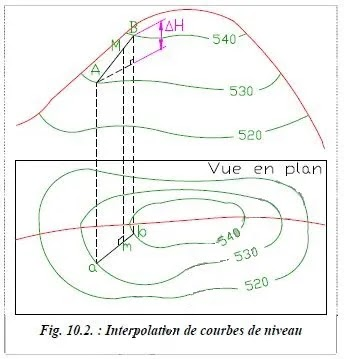Understanding simple difinition contour lines ₪ 1.1. Principe of interpolation

# Understanding simple difinition contour lines ₪ 1.1. Principe of interpolation

Understanding interpolation allows you to wisely choose the number and passion of the points to be surveyed.

The altitude at point M Located between the contour lines
530 and 540 is determined by considering the constantly sloping ground between and B.
Points and B are the closest points to on contour lines 530 and 540; here ΔH 10 m.

The slope at point M is : p ΔH
ab
The distance ab is the real distance, that is to say the distance measured on the plan and divided by the scale of the plan.
The altitude of M is : HM  = HA + am  ΔH
abThis last formula can be applied with the distances measured on the plan; the scale factor simplifies.